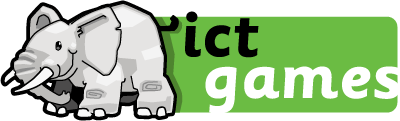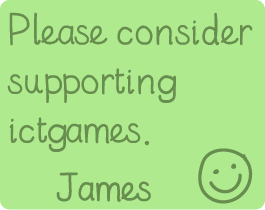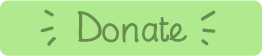# A Different Shop (a shop with a difference!)

### Solve word problems using subtraction as 'difference'

A game based on the hardest* questions in previous Year 2 SATs Reasoning papers.

• Year 1: 1NF: Develop fluency in addition and subtraction facts within 10.
• Year 2: 2AS: Add and subtract across 10.
• Year 2: 2AS: Recognise the subtraction structure of 'difference' and answer questions of the form, "How many more...?"
• Year 2: 2AS: Add and subtract within 100 by applying related one-digit addition and subtraction facts: add and subtract only ones or only tens to/from a two digit number.
• Year 2: 2AS: Add and subtract within 100 by applying related one-digit addition and subtraction facts: add and subtract any 2 two digit numbers.
• Year 3: 3AS: Manipulate the additive relationship: Understand the inverse relationship between addition and subtraction, and how both relate to the part–part–whole structure. Understand and use the commutative property of addition, and understand the related property for subtraction.

### Instructions:

2. Click on the items mentioned in the word problem. This will show you their price.
3. Click on the think bubble. Look at the Bar Model and use it to help you find the maths. What do you need to do?
5. After you've completed 5 problems the game will randomly thow in questions dealing with giving change.
6. The game finishes after you have completed 20 word problems.
7. Levels 4 and 5 are two-part questions. Extra tricky!! You're buying two items and then finding the difference.

Have fun!Made using theandlibraries.